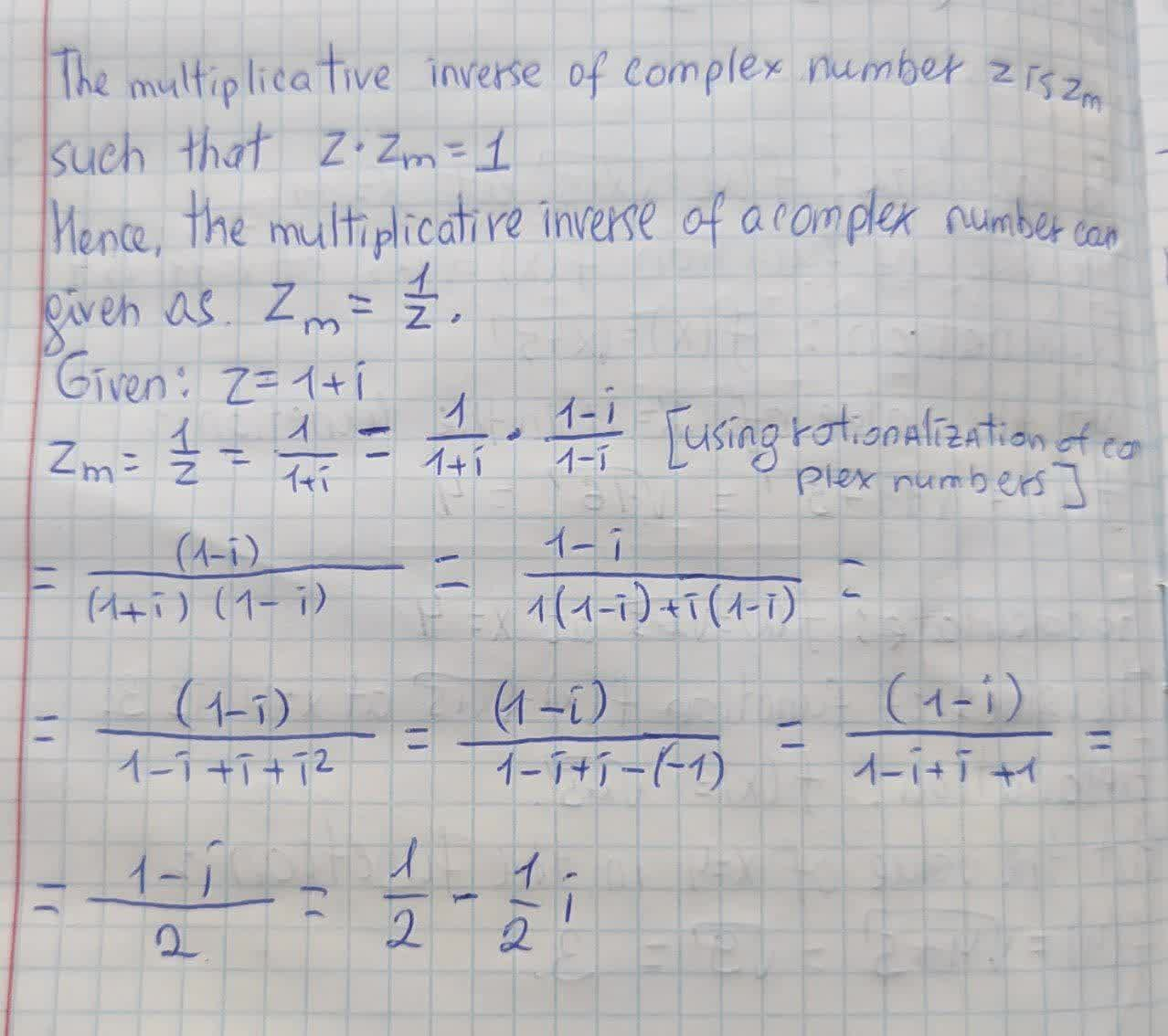# Multiplicative Inverse of a Complex Number The multiplicative inverse of aJoni Kenny 2021-08-16 Answered
Multiplicative Inverse of a Complex Number The multiplicative inverse of a complex number z is a complex number zm such that $$\displaystyle{z}\times{z}{m}={1}$$. Find the multiplicative inverse of complex number.
$$\displaystyle{z}={1}+{i}$$

• Questions are typically answered in as fast as 30 minutes

### Plainmath recommends

• Get a detailed answer even on the hardest topics.
• Ask an expert for a step-by-step guidance to learn to do it yourself.stuth1

Solution is on photo